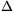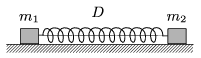Mathematical and Physical Journal
for High Schools
Issued by the MATFUND Foundation
 Already signed up? New to KöMaL?

#Problem P. 3866. (February 2006)

P. 3866. Two small bodies of masses m1, and m2 are attached to the two ends of an ideal spring of spring constant D. The spring is stretched byl, and then the two bodies are released at the same time. Find the greatest speeds of the two masses, the amplitudes of their oscillations, and the frequencies. Friction is negligible. (Data: m1=1 kg, m2=2 kg, D=100 N/m,l=9 cm.)(4 pont)

Deadline expired on March 13, 2006.

### Statistics:

 138 students sent a solution. 4 points: 71 students. 3 points: 17 students. 2 points: 14 students. 1 point: 22 students. 0 point: 14 students.

Problems in Physics of KöMaL, February 2006